## If Cell is This Value or That Value

IF function is frequently used in Excel worksheet to return you expect “true value” or “false value” based on the result of logical test. If you want to see if a cell is A or B, and if one of them is true, true value is returned and if none of them is true, false value is returned. It seems that we can build a formula with IF function to solve this problem because in this case there are two results “if true value” and “if false value”, and the final result is retrieved from the two results based on the logical test result. But, unlike normal IF formula, there are two logical tests here “cell=A” or “cell=B”, so except IF function, we also use OR function inside IF function to solve this problem. See example below.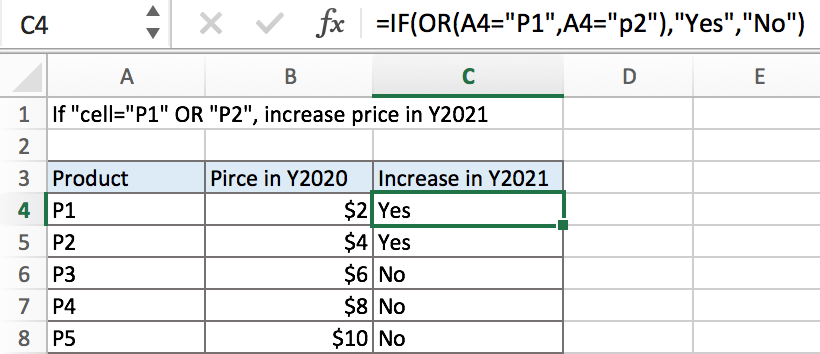In above example, in product list, if cell is “P1” or “P2”, its price will increase in a new year, we will mark “Yes” for them in “Increase in Y2021” list. Otherwise, if cell is not “P1” or “P2”, the price will not increase in Y2021, we will mark “No” in “Increase in Y2021” list. IF function can handle this case effectively, it returns either “Yes” or “No”.

## FORMULA

To test if cell equals a certain text string, the generic formula is:

=IF(OR(A1=”A”,A1=”B”),”true value”,”false value”)

Formula in this example:

=IF(OR(A4=”P1″,A4=”P2″),”Yes”,”No”)

## EXPLANATION

OR function is often used as logical test part inside IF function. It can test multiple logical conditions at one time, and if one of the multiple conditions is true, it returns true. In this example, if A4=”P1” is true, or A4=”P2” is true, OR delivers TRUE to IF function.

Syntax: OR (logical1, [logical2], …)

IF function allows you to create a logical comparison between your value and reference value (for example “A1>0”), and set true value and false value what you expect to return as test results. IF function returns one of the two results based on logical comparison result.

Syntax: IF(logica_test,[value_if_true],[value_if_false])

In this example, we want to mark “Yes” for those cells contain “P1” or “P2”. In normal situation, there is only one logical condition in IF formula, for example IF(cell=”P1”,”Yes”,”No”), but there are two conditions cell=”P1” and cell=”P2”, IF returns true value is one of them is true. As this is a OR relationship, so we add OR function inside IF, and IF logical test is consisted of OR function with the two logical conditions: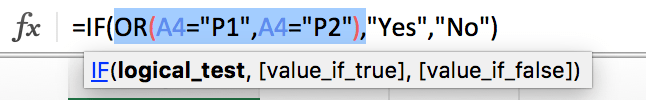To test if A4 is P1 or P2 or not matched, we create the logical comparison OR(A4=”P1″,A4=”P2″):

• The symbol = is a logical operator that means “equal to”
• P1 and P2 are enclosed in double quotes “” as they are texts
• Double quotes “” should not be ignored in this case. If missing double quotes, Excel will prompts warning message that the formula you typed contains an error, if you ignore the error, #VALUE! is displayed in cell

After running the logical test, IF function retrieves true or false result based on the test result. In this example, for the two arguments “value_if_true” and “value_if_false”, we set “Yes” as true result and “No” as false result. So the formula is: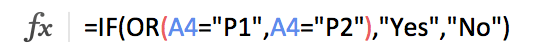In this example, A4 is P1, logical test result is true, so IF function returns True value “Yes”.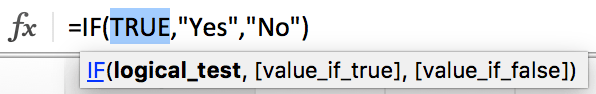For B6 which records P3, it cannot match either of the two conditions, so OR function returns False and deliver false result to IF function, IF retrieves False value “No” from the two results.

In IF, true value and false value can be set as empty string “”, a text, a number or a formula, you can set what you expect to these two values.

## MORE COMPLICATED EXAMPLE

In example below, if product is P1 or P2, price in Y2021 should be increased by 20%. So, if the logical test is true, true value is current price*1.2, as old price is saved in B4, so we set B4*1.2 as true value. If logical test is false, there is no need to update price, so keep original price in B4 as new price, set B4 as false value. In this example, true value is a formula, and false value is a cell reference.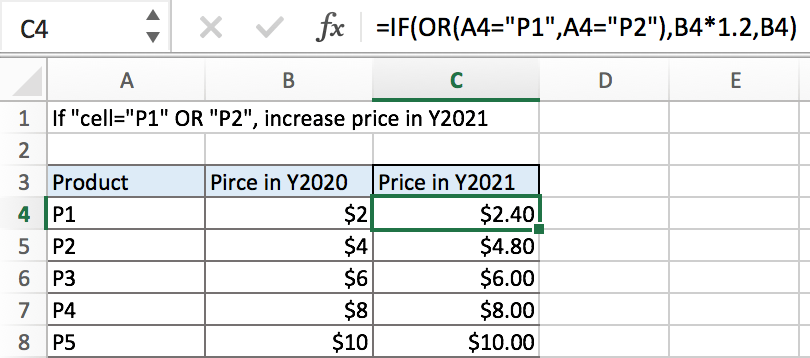### Related Functions

• Excel IF function
The Excel IF function perform a logical test to return one value if the condition is TRUE and return another value if the condition is FALSE. The IF function is a build-in function in Microsoft Excel and it is categorized as a Logical Function.The syntax of the IF function is as below:= IF (condition, [true_value], [false_value])….
• Excel OR Function
The Excel OR function used to test multiple conditions and returns TRUE if any of the conditions are TRUE.The syntax of the OR function is as below:=OR(logical1, [logical2], …)…

## Determine If a Date Falls on the Weekend

This post will guide you how to determine if a date falls on the weekend in Excel. How do I check if a date falls on the weekend with a formula in Excel. How to check if a date is a weekend in Excel.

## Check if a Date Falls on the Weekend

Assuming that you have a list of data that contain dates in range A1:A5, and you want to check each date if it falls on the weekend. How to achieve it. You can create a formula based on the IF function, the OR function and the WEEKDAY function. Just like this:

`=IF(OR(WEEKDAY(A1)=1,WEEKDAY(A1)=7),TRUE,FALSE)`

Type this formula into Cell B1, and press Enter key in your keyboard, and then drag the AutoFill Handle from Cell B1 to B5.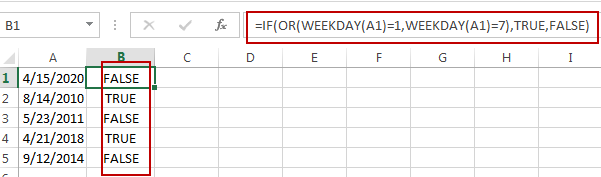This formula will check if a date falls on the weekend, it will return TRUE, otherwise, it will return FALSE.

### Related Functions

• Excel IF function
The Excel IF function perform a logical test to return one value if the condition is TRUE and return another value if the condition is FALSE. The IF function is a build-in function in Microsoft Excel and it is categorized as a Logical Function.The syntax of the IF function is as below:= IF (condition, [true_value], [false_value])….
• Excel WEEKDAY function
The Excel WEEKDAY function returns a integer value representing the day fo the week for a given Excel date and the value is range from 1 to 7.The syntax of the WEEKDAY function is as below:=WEEKDAY (serial_number,[return_type])…
• Excel OR Function
The Excel OR function used to test multiple conditions and returns TRUE if any of the conditions are TRUE.The syntax of the OR function is as below:=OR(logical1, [logical2], …)…

## Excel OR Function

This post will guide you how to use Excel OR function with syntax and examples in Microsoft excel.

### Description

The Excel OR function used to test multiple conditions and returns TRUE if any of the conditions are TRUE. Otherwise, it will return FALSE. You can use the OR function as the logical test inside the IF function and it also can be combined with the AND function to create a new Excel Formula.

The OR function is a build-in function in Microsoft Excel and it is categorized as a Logical Function.

The OR function is available in Excel 2016, Excel 2013, Excel 2010, Excel 2007, Excel 2003, Excel XP, Excel 2000, Excel 2011 for Mac.

### Syntax

The syntax of the OR function is as below:

`=OR(logical1, [logical2], ...)`

Where the OR function arguments are:

• logical1 – This is a required argument.  The first condition or logical value that you want to test.
• logical2 – This is an optional argument. The second condition or logical value that you want to test. And it can be up to 255 logical test argument in Excel 2007 or later. And it only can up to 30 arguments in excel 2003.

### Excel OR Function Examples

The below examples will show you how to use Excel OR Function to test a number of supplied conditions and return TRUE or FALSE.

1# To return TRUE if B1 is greater than 1 or less than 10, otherwise returns FALSE, using the following formula:

=OR(B1>1,B1<10)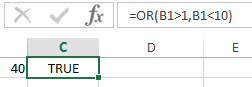2# to return the value in Cell B2 if it is greater than 1 or less 10, otherwise returns the message “this is not a value”, using the following formula:

=IF(OR(B1>1,B1<10), B2, “this is not a value”)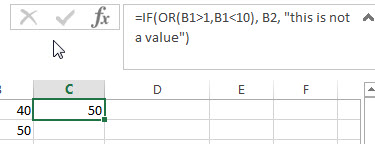Related Functions

• Excel AND function
The Excel AND function returns TRUE if all of arguments are TRUE, and it returns FALSE if any of arguments are FALSE.The syntax of the AND function is as below:= AND (condition1,[condition2],…) …
• Excel IF function
The Excel IF function perform a logical test to return one value if the condition is TRUE and return another value if the condition is FALSE….

### Related Formulas

• Excel IF formula with NOT logical function
If you want to check several test conditions in an excel formula,then take different actions. You can use NOT function in combination with the AND or OR logical function in excel IF function….
• Excel IF formula with OR logical function
If you want to check if one of several conditions is met in your excel workbook, if the test is TRUE, then you can take certain action. You can use the IF function combining with OR function to construct a condition statement…
• Excel IF formula with AND & OR logical functions
If you want to test the result of cells based on several sets of multiple test conditions, you can use the IF function with the AND and OR functions at a time…

## Excel IF formula with operator : greater than,less than

This post will guide you how to use if function with “greater than”,  “greater than or equal to”, ”less than” and “less than or equal to” logical operators in excel.

## Greater than, Greater than or equal to, Less than, less than or equal to

The “greater than”(>)operator will compare the size of two different values in cells and then return TRUE if the first value in cells compared is larger than the second values in cells. FALSE if they are not.

The “greater than or equal to” (>=) operator will return TRUE if the first value in cells is larger than the second or if the two values are equal.

The “Less than” operator returns TRUE if the first value in cell is smaller than the second value in cells.

The “Less than or equal to” operator returns TRUE if the first value in cell is smaller than the second of the two values are equal.

Those excel comparison operators mostly used to compare numbers, date and time values.

Also, we can use those operators to compare text string, and it will compare the value of the first letters in the text string. And if the first letters are same, it will compare the second letters and so on.

Let’s see the below excel if formula with greater than operator:

 Formula example Description =A1>10 Returns True if a number value in cell A1 is greater than 10, FALSE if they are not. =A1>=(B1*2) Returns True if a number value in cell A1 is greater than or equal to the value of  B1*2, FALSE if they are not. =A1

## The Generic IF formula with Greater than operator

Now we can use the above logical test with greater than operator. Let’s see the below generic if formula with greater than operator:

`=IF(A1>10,”excelhow.net”,”google”)`

If you want to take the different action when a value in cells is greater than a certain value (it can be number, text, date or another function), then you can use the IF function with greater than operator to make a logical test and return one value if the logical test condition is true, or return another value if the logical test condition is false.

For the above IF formula, it just tests the value in cell A1 to check if the value is greater than 10. If TRUE, the IF formula will return “excelhow.net”. If FALSE, the IF function will return “google”.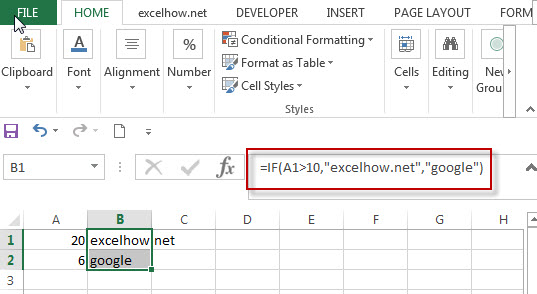You might want to return “excelhow.net” if the test condition is TRUE. Or return nothing if the test returns FALSE. You can use an empty string (“”) as False value in the IF function, see below if formula:

`=IF(A1>10,”excelhow.net”,””)`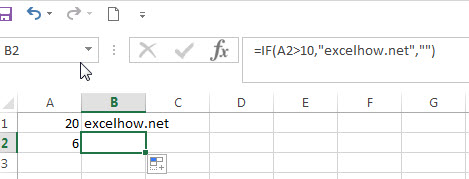## The Generic IF formula with Greater than or equal to operator

`=IF(A1>=(B1*2),”excelhow.net”,”google”)`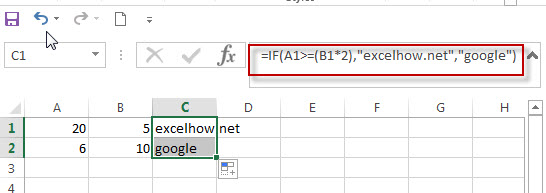## The Generic IF formula with less than operator

`=IF(A1<DATEVALUE(“10/30/2018”),”excelhow.net”,”google”)`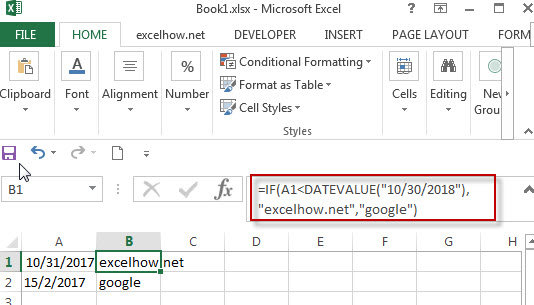## The Generic IF formula with Greater than operator

`=IF(A1<=”excelhow”,”excelhow.net”,”google”)`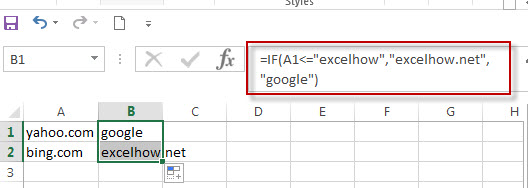### Related Functions

• Excel AND function
The Excel AND function returns TRUE if all of arguments are TRUE, and it returns FALSE if any of arguments are FALSE.The syntax of the AND function is as below:= AND (condition1,[condition2],…) …
• Excel IF function
The Excel IF function perform a logical test to return one value if the condition is TRUE and return another value if the condition is FALSE….
• Excel nested if function
The nested IF function is formed by multiple if statements within one Excel if function. This excel nested if statement makes it possible for a single formula to take multiple actions…
• Excel DATEVALUE Function
The Excel DATEVALUE function returns the serial number of date. And it can be used to convert a date represented as text format into a serial number that recognizes as a date format.The syntax of the DATEVALUE function is as below:=DATEVALUE(date_text)…

## Excel IF formula with AND & OR logical functions

If you want to test the result of cells based on several sets of multiple test conditions, you can use the IF function with the AND and OR functions at a time.

Assuming that you have the following test logic to check the exam scores:

Logic test1: 60<=B1<=70

Logic test2: 60<=C1<=70

If any of the above conditions are met, then mark the result as “good”, otherwise, mark the result as “bad”. We need to use two AND functions to reflect the above two logical test and enclose them in the OR function as one logical test in the Excel IF formula. Let’s write down the below IF formula with AND & OR logical functions:

`=IF(OR(AND(B1>=60,B1<=70),AND(C1>=60,C1<=70)),"good","bad") `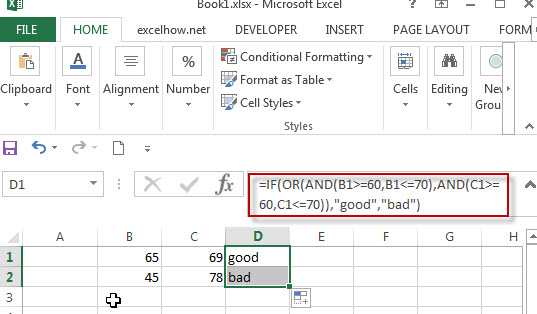Let’s see another IF formula with AND and OR functions as follows:

`=IF(AND(B1="tom",OR(C1>=60,D1>=60)),"pass","")`

When B1 is equal to “tom”, and either C1 is greater than or equal to “60” or D1 is greater than or equal to 60, the formula returns “pass”. Otherwise, the formula will return an empty string.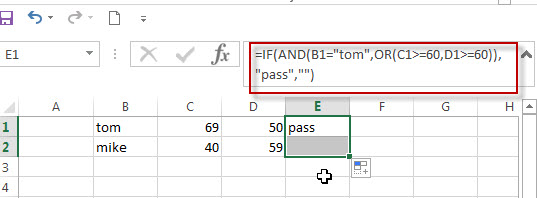If we just want to check the cells where the value in column B is “tom” AND the score in column C is greater than or equal to 60. If you want to return TRUE, you can use the below logical test statement with the AND function:

`AND(B1="tom",C1>=60)`

Next, we can extend the above statement, we want to check the cells if the values in column B is “tom” AND the scores in column C or column D is greater than or equal to 60. Then we can nest the OR function in the above AND function like below:

`AND(B1="tom",OR(C1>=60,D1>=60))`

### Related Formulas

• Excel IF formula with equal to logical operator
The “Equal to” logical operator can be used to compare the below data types, such as: text string, numbers, dates, Booleans.  This section will guide you how to use “equal to” logical operator in excel IF formula with text string value and dates value…
• Excel IF formula with OR logical function
If you want to check if one of several conditions is met in your excel workbook, if the test is TRUE, then you can take certain action. You can use the IF function combining with OR function to construct a condition statement…
• Excel IF formula with AND logical function
You can use the IF function combining with AND function to construct a condition statement. If the test is FALSE, then take another action…

### Related Functions

• Excel AND function
The Excel AND function returns TRUE if all of arguments are TRUE, and it returns FALSE if any of arguments are FALSE.The syntax of the AND function is as below:= AND (condition1,[condition2],…) …
• Excel OR function
The Excel OR function used to test multiple conditions and returns TRUE if any of the conditions are TRUE. Otherwise, it will return FALSE. The syntax of the OR function is as below:=OR(logical1, [logical2], …)…
• Excel IF function
The Excel IF function perform a logical test to return one value if the condition is TRUE and return another value if the condition is FALSE….
• Excel nested if function
The nested IF function is formed by multiple if statements within one Excel if function. This excel nested if statement makes it possible for a single formula to take multiple actions…

## Excel IF formula with OR logical function

This post will guide you how to use IF function combining with OR logical function in Excel.

If you want to check if one of several conditions is met in your excel workbook, if the test is TRUE, then you can take certain action. You can use the IF function combining with OR function to construct a condition statement. If the test is FALSE, then take another action.

## Excel formula using IF & OR function

The syntax of OR function in excel is as follow:

`=OR(condition1,[condition2],...)`

The Excel OR function returns TRUE if any of the conditions are TRUE in logic test. Otherwise, it returns FALSE.

Now we want to check the results of two exam scores where the first score in cell A1 is greater than or equal to 40 OR the second score in cell B1 is greater than or equal to 50. If any of conditions are TRUE, then returns the text of “good”, and if FALSE returns “bad”.

Based on the above logic, we can write down the below IF formula:

`=IF(OR(A1=”40”,B1=”50”),”good”, ”bad”)`

We can enter the above formula into cell D1, and then drag the Fill Handle to the range D1:D3.it will apply for the other cells for this formula.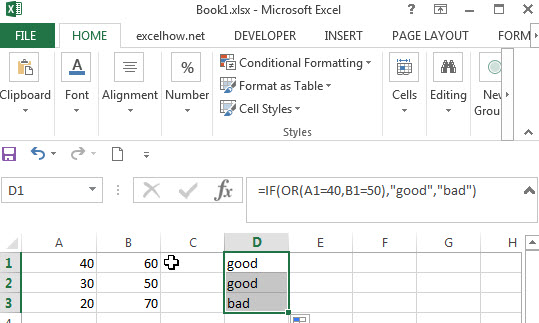So the difference from the IF&AND formula discussed in the previous post is that the IF&OR formula will return TRUE if any of conditions are true.

In the above formula, we just want to check both A1 and B1 cells, the Excel will take an action when the result of test condition is TRUE. In this case, we will add “good” text in the D1 cell. If the test condition is FALSE, assuming that you do not want to display anything in the cell D1, then we can write down the following excel if formula:

`=IF(OR(A1=”40”,B1=”50”),”good”,””)`

Normally, you can use as many AND/OR logical functions as your logic requires, but there is limitation for argument numbers, we can follow the below limitation for excel formula:

1. No more than 255 arguments in Microsoft excel 2016,2013,2010 and 2007
2. No more than 8192 characters in Microsoft excel 2016,2013,2010 and 2007
3. No more than 30 arguments and the length of formula should not exceed 1024 characters in Microsoft excel 2003 or lower version.

### Related Functions

• Excel OR function
The Excel OR function returns TRUE if any of the conditions are TRUE in logic test. Otherwise, it returns FALSE.
• Excel AND function
The Excel AND function returns TRUE if all of arguments are TRUE, and it returns FALSE if any of arguments are FALSE.The syntax of the AND function is as below:= AND (condition1,[condition2],…) …
• Excel IF function
The Excel IF function perform a logical test to return one value if the condition is TRUE and return another value if the condition is FALSE….
• Excel nested if function
The nested IF function is formed by multiple if statements within one Excel if function. This excel nested if statement makes it possible for a single formula to take multiple actions…

## Excel Nexted IF Functions (Statements) Tutorial (15 IF Formulas examples)

This tutorial will guide you how to use nested Excel IF function  (include multiple If statements in excel formula) with syntax and provide about 15 nested IF formula examples with the detailed explanation in Microsoft excel.

## Description

The Excel IF function perform a logical test to return one value if the condition statement is TRUE and return another value if the condition statement is FALSE. The IF function is a build-in function in Microsoft Excel and it is categorized as a Logical Function.

The excel if function just only test one condition and if you want to deal with more than one condition and return different actions depending on the result of the tests,  then you need to include several IF statements (functions) in one excel IF formula, these multiple IF statements are also called Excel Nested IF formula(Nested IFs). It’s also similar with IF-THEN-ELSE statement.

The nested IF function is formed by multiple if statements within one Excel if function. This excel nested if statement makes it possible for a single formula to take multiple actions.

The most recent versions of Excel (Excel 2016, Excel 2013, Excel 2010 and Excel 2007) allow 64 IF functions (statements) in one formula, and it was only possible up to 7 nested IF functions (statements) in Excel 2003 and lower version.

## Syntax

The syntax of Nested IF function is as below:

`=IF(Condition_1,Value_if_True_1,IF(Condition_2,Value_if_True_2,Value_if_False_2))`

Where the Nested IF function argument is:

Condition_1 –  The condition that you want to test  in the first IF statement.

Value_if_True_1 – The value that is returned if first IF statement is True. If the condition_1 return False, then move into the next IF function.

Condition_2 – The condition that you want to test in the second IF statement.

Value_if_True_2 – The value that is returned if second IF statement is True.

Value_if_False_2 – The value is returned if second IF statement is False.

This is equivalent to the following IF THEN ELSE statement:

```IF Condition_1 THEN
Value_if_True_1
ELSEIF Condition_2
Value_if_True_2
ELSE
Value_if_False_2
END IF```

## Examples of Nested IF function (Statement) in Excel

The below examples will show you how to use Excel Nested IF function with the detailed explanation of their syntax and logic.

Example 1#  The most basic Nested IF function with one level of nesting

If you want to write a nested if function to test the following calculation logic for assigning value in the cell A1.

```IF A1 =="excelhow" THEN
return "excel"
ELSEIF A1 == "excelhow.net" THEN
return "MS excel"
ELSE
return "MS"
END IF```

we can write a nested IF function based on the above logic as follows:

`=IF(A1="excelhow", "excel", IF(A1="excelhow.net","MS excel","MS"))`

In above Nested IF formula, the nested if function is shown with bold style and it is inside the outer IF function. we can see that if A1 is not equal to the “excelhow“, then the second nested  IF function will be test. and if second IF condition statement return FALSE, then the entire IF function will return “MS” value.

Example 2#  The Nested IF function with two levels of nesting

Assuming that you want to test more than one condition statement in the above nested if function, add one condition to test if the value of the cell A1 reference is equal to “www.excelhow.net” , If TRUE, then return “Microsot excel“.

The calculation Logic is as below:

```IF A1 =="excelhow" THEN
return "excel"
ELSEIF A1 == "excelhow.com" THEN
return "MS excel"
ELSEIF A1 == "www.excelhow.net" THEN
return "Microsoft excel"
ELSE
return "MS"
END IF```

we can add one more IF statement inside the second IF function in the above excel nested if formula in example1. let’s see the below nested if function with tow level nesting:

`=IF(A1="excelhow", "excel", IF(A1="excelhow.com","MS excel",IF(A1="www.excelhow.net","Microsoft excel","MS")))`

In the above nested excel IF formula, the first nested if function is marked with red color, and the second nested excel if function is marked with blue color.

If the both first and second conditions are False and the third IF condition will be check, IF A1 is equal to “www.excelhow.net” , then return “Microsoft excel“, or the entire nested IF formula will return “MS“.

Example 3#  Describes the each IF function contained in the nested IF function

​We will use one typical example of excel nested if function to describe each IF function included in the nested if function.

Assuming that you need to assign a grade based on a score with the following test conditions:

Let’s write a nested if function based on the above logic as follows:

`=IF(A1>=80, "excellent", IF(A1>=60, "good", IF(A1>0, "bad", "no valid score")))`

For the above excel if formula, lets describe it for each IF function statement.

1# IF Cell A1 is greater than or equal to 80, then the formula will return “excellent” or move to the second If function.

2# If Cell A1 is greater than or equal to 60, then the formula will return  “good” or move to the third IF function

3# IF Cell A1 is greater than 0, then the formula will return “bad”, or the IF function will return “no valid score”.

Example 4#  Describes each If function in the excel Nested IF Statement (another simple example of if function)

Let’s describe the below Nested IF Function example:

`=IF(A1<=6,60, IF(A1<=8,80,IF(A1<=10,100,200)))`

a) If cell A1 is equal to 6 or less than 6, then return value 60 in cell C1. Let’s see below screenshot.

b) If Cell A1 is greater than 6 and less or equal to 8, then retrun value 80 in Cell C1.

c) If cell A1 is greater than 8 and less than or equal to 10, then return value 100 in cell C1.

d) If cell A1 is greater than 10 , then the Nested if function will return the last value “200”in cell C1.

Example 5#  Excel Nested IF function with arithmetic operator (+, -, * , /)

Assuming that you want to write a Nested If function to reflect the following logic tasks:

a) IF Cell A1 is less than 10, then multiply by 10.

b) IF Cell A1 is greater than or equal to 10 but less than 20, then add 20

c) IF Cell A1 is greater than or equal to 20 but less than 30, then minus 20

d) IF Cell A1 is greater than or equal to 30 but less than 50, then divided by 20

The nested IF formula is as follows:

`=IF(A1<10,A1*10,IF(A1<20,A1+20,IF(A1<30,A1-20,IF(A1<50,A1/20))))`

a1) if Cell A1 is less 10 (A1=5), then the first If condition matched and will take multiply action, A1 * 10=5*10=50, so it will return 50 in the cell C1

b1) if Cell 10<=A1<20 (A1=15), then the second if condition matched and will take add action, A1+20=15+20=35, so it will return 35 in the cell C1.

c1) if Cell 20<=A1<30(A1=25), then the third if condition matched and will take minus action, A1-20=25-20=5, so it will return 5 in the cell C1.

d1) if Cell 30<=A1<50 (A1=35), then the forth if condition matched and will take divide action, A1/20=35/20=1.75, so it will return 1.75 in the cell C1.

Example 6#  Excel Nested IF function with logical function –AND

Assuming that you need a nested if function to reflect the following logic:

a) IF A1+B1 is less than 10, then return 10

b) IF A1+B1 is greater than 10 but less than or equal to 20, then return 20

c) IF A1+B1 is greater than 20 but less than or equal to 30, then return 30.

d) IF A1+B1 is greater than 30, then return 200.

Let’s write the following nested IF formula in the cell C1:

`=IF(A1+B1<10,10, IF(A1+B1<=20,20, IF(A1+B1<=30,30,200)))`

The above formula just use basic nested IF function syntax, we also can use logic function to re-write it, the nested if formula with AND function is as follows:

`=IF((A1+B1)<10,10,IF(AND((A1+B1)>10,(A1+B1)<=20),20, IF(AND((A1+B1)>20,(A1+B1)<=30),30,200)))`

The above nested IF formula combined with two AND function.

In the second IF Statement, AND((A1+B1)>10,(A1+B1)<=20) will check if 10<A1+B1<=20, If TRUE, then the formula will return 20.

In the third IF Statement, AND((A1+B1)>20,(A1+B1)<=30) will check if 20<A1+B1<=30, If TRUE, then the formula will return 30.

Example 7# Excel Nested IF function with logical function –OR

Assuming that you need a nested if function to reflect the following logic:

a) IF Cell A1=5, return A1/B1

b) IF Cell A1=10, return A2/B2

c) IF Cell A1=15 or A1=20, return A3/B3

In Cell C1, we can write the below nested if formula based on the above conditions.

`=IF(A1=5,A1/B1,IF(A1=10,A2/B2,IF(OR(A1=15,A1=20),A3/B3)))`

One OR function be used in the above excel nested if function, it will check if A1=15 or A1=20, if TRUE, then return A3/B3.

Example 8#  Excel nested if function with text and logical function AND

Wrote a nested if function with text to reflect the following logic:

a) If Cell A1=”E” and Cell B1=5, then return “Excel”

b) If Cell A1=”P” and Cell B1=3, then return “PPT”

c) If Cell A1=”W” and Cell B1=4, then return “Word”

d) Else return “Access”

In Cell C1, try to enter into the following excel nested If formula with AND function:

`=IF(AND(A1="E",B1=5),"Excel", IF(AND(A1="P",B1=3),"PPT", IF(AND(A1="W",B1=4),"Word","Access")))`

Example 9#  Excel nested if function with ISBLANK function and logical function AND

a) If you want to wrote a nested if function with ISBLANK function and logical function AND to reflect the following logic:

b) If both Cell A1 and Cell B1 are empty, then return “”

c) If only Cell A1 is empty, then return B1-today()

d) If both two cells A1 and B1 are not empty, then return “excel” string.

In Cell C1, use the following excel nested If formula with ISBLANK and AND function:

`=IF(AND(ISBLANK(A1),ISBLANK(B1)),"",IF(ISBLANK(A1),B1-TODAY(),"excel"))`

Example 10#  Using nested IF functions to check grade level based on student’s score(multiple IF statements)

The logic is as below:

We will write a nested If function that reflect the above logic, and will check if the score is below 50, If TRUE, it is considered as “Fail”. If FALSE, move into the next IF statement to test if the score is between 51 and 60 and it is considered as “Grade C”. If False, we will move into another IF statement to check if the score is between 61 and 80, IF True and it is considered as “Grade B”. If FASLSE, just check the rest conditions.

We can use a nested if formula as follows:

`=IF(B2<=50,"Fail",IF(AND(B2>=51, B2<=60), "Grade C", IF(AND(B2>=61, B2<=80),"Grade B",IF(AND(B2>=81,B2<=95),"Grade A", "Grade A+"))))`

Example 11#  Nested IF function for checking two Empty Cells

Let’s see the below image a product table of a company (need to create an excel table firstly):

a) If we need to check both “Price” cell and “Quantity” cell are empty, If True, then return empty. If the only “price” cell is empty only, IF True, return empty.

b) If the only “Quantity” cell is empty, IF True, return empty.

c) If both “price” and “Quantity” are not empty, then return multiply Price * Quantity as subtotal value.

So To check both “Price” and Quantity cells, we can use table header name as condition variable to test each Price cells or Quantity cells, so we can write the nested if formula as follows:

`=IF([Price]="","",IF([Quantity]="","",[Price]*[Quantity]))`

Just using the above excel if formula in the subtotal cells, the formula will check the first IF statement if Price Cell is empty, IF TRUE, then will return empty (“”) in the subtotal cell. IF FALSE, then move to the next IF statement and so on. Last, IF neither cell is empty, then will return the value of multiply [Price]*[Quantity] in subtotal cell.

Of course, we can use another nested if function to achieve the above calculation logic (easy to understand).

`=IF(ISBLANK(B2),"",IF(ISBLANK(C2),"", B2*C2))`

OR

`=IF(B2="","",IF(C2="","", B2*C2))`

## Nested IF Functions Order

There is one important thing that need us keep in mind when write Excel Nested IF Function, it is the order of nested IF function. It can nested up to 64 If statements, and how to order multiple IF condition statements, it is key point. Or the wrong result will be returned. The point is that excel nested if function will test the first if condition in the order, once any condition is met, and the subsequent if conditions will not be checked.

So let’s remember the below rules while writing excel nested if function:

• The most important condition First or Harder Test First

Let’s see the below example what it means:

Example 12#

There are two test conditions in the following excel nested if function:

`=IF(B1>=90,"excellent",IF(B1>=60,"good","bad"))`

When using this formula in the cell B3, If the amount in cell B1 is 95, then “excellent” would be returned. because it is greater than 90. And the second IF condition will not be evaluated.

However, if the order of nested if statment are reversed as follows:

`=IF(B1>=60,"good",IF(B1>=90,"excellent","bad"))`

The above formula would check for the condition B1>=60 first, if the amount in cell B1 is 95,  then the value “good” would be returned in cell B3. Because the Cell B1 match the first test condition, and it will not check the second if condition and will return the incorrect result.

## Nested IF Function Alternatives

To make your excel formulas more efficiency and fast, you can try to use the following alternatives to excel nested if function.

1)
Excel nested if function can be easily replaced with the VLOOUP, Lookup, INDEX/MATCH or CHOOSE functions.

Example 13#  Use VLookup function instead of nested IF function

Nested IF function:

`=IF(D1=39,"excelhow.net", IF(D1=45,"two",IF(D1=34,"google.com","")))`

Vlookup function:

`=VLOOKUP(39,A1:B3,2,FALSE)`

Example 14#  Use CHOOSE function instead of nested if function

Nested If unction:

`=IF(B1>=60,"good","bad")`

Using CHOOSE function as follows:

`=CHOOSE(IF(B1<60,1,2),"bad","good")`

2) Use IFS instead of nested if function

3) Use the CONCATENATE function or the concatenate operator (&).

Example 15#

Nested IF function:

`=IF(B1="A","excellent",IF(B1="B","good","bad"))`

Using CONCATENATE function as follows:

`=CONCATENATE(IF(B1=”A”,”excellent”,””),IF(B1=”B”,”good”,””),IF(B1=”C”,”bad”))`

Question1: Is there any tool to help write Excel formulas and nested Ifs?

This is an Excel formula with nested IF statements:

`=IF((B2="East"),4,IF((B2="West"),3,IF((B2="North"),2,IF((B2="South"),1,""))))`

To essentially accomplish this:

```If cell B2 = "East"
return "4"
ElseIf cell B2 = "West"
return "3"
ElseIf cell B2 = "North"
return "2"
ElseIf cell B2 = "South"
return "1"
Else
return ""```

Can Excel formulas be written in such a “more readable” manner and converted to the official syntax? Is there any tool to help write Excel formulas?

Answer: Excel Formula Formatter add-in by Rob van Gelder, mentioned at Daily Dose of Excel.

Excel’s formula bar ignores line feeds and white space, so you can Alt+Enter and spacebar to format the formulas however you like. I’ve tried it and I quickly stopped doing it. Too much spacebar-ing, especially if you need to edit.

Question2: I am working on an excel file, and i am trying to use a nested if formula to achieve what i would like.

i have two columns:A B.  And the condition is this: if the value in a2=a3, then check if the minus of b2 and b3 is certain value, and if it is, put a yes, else put a no. this will iterate till the end of the excel file.

so far here is what i have. not sure how to use the excel formulas. any help is much appreciated.

`if(a2=a3,b2-b3=5 or b2-b3=-5 or b2-b3=20 or b2-b3=-20, "yes", "no")`

Answer: you should be able to use the OR function within your nested if formula to test for “B2-B3=5,B2-B3=-5,B2-B3=20,B2-B3=-20” as follows:

`=IF(A2=A3,IF(OR(B2-B3=5,B2-B3=-5,B2-B3=20,B2-B3=-20),"yes","no"),"no")`

### Related Functions

• Excel IF function
The Excel IF function perform a logical test to return one value if the condition is TRUE and return another value if the condition is FALSE….
• Excel And function
The Excel AND function returns TRUE if all of arguments are TRUE, and it returns FALSE if any of arguments are FALSE…
• Excel Concat Function
The excel CONCAT function combines 2 or more strings or ranges together. The syntax of the CONCAT function is as below: =CONCAT (text1,[text2],…) …
• Excel Vlookup Function
The Excel VLOOKUP function lookup a value in the first column of the table and return the value in the same row based on index_num position.The syntax of the VLOOKUP function is as below:= VLOOKUP (lookup_value, table_array, column_index_num,[range_lookup])…
• Excel Choose Function
The Excel CHOOSE function returns a value from a list of values. The CHOOSE function is a build-in function in Microsoft Excel and it is categorized as a Lookup and Reference Function.The syntax of the CHOOSE function is as below:=CHOOSE (index_num, value1,[value2],…)…
• Excel ISBLANK function
The Excel ISBLANK function returns TRUE if the value is blank or null.The syntax of the ISBLANK function is as below:= ISBLANK (value)…